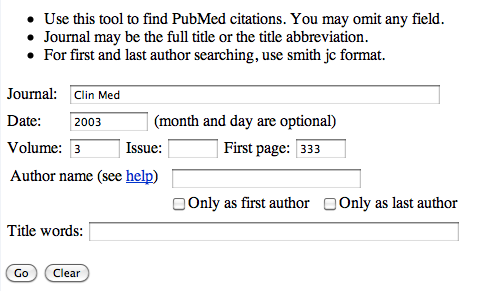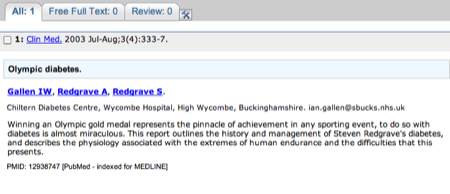## Tuesday 6 October 2009

### Finding scientific references given in talks or lectures

I have been asked how you can track down papers online when a lecturer gives a paper reference as, for example:

Clin Med. (2003) 3, 333-7

The easiest way to track this down is to use 'Single Citation Matcher'.

http://www.ncbi.nlm.nih.gov/corehtml/query/static/citmatch.html

On the page enter the required information, so using the above reference, Clin Med. (2003) 3, 333-7, you should end up with:Click the 'Go' button and you should be taken to the paper (and any link to the full paper if available).The abstract for the paper - don't forget there may be a link to the full paper on the far righthand side of the page.

## Monday 5 October 2009

### Protein sequence to DNA - Degeneracy calculation

I have had a some questions about reverse translation of protein to DNA, and degeneracy....

It is 20 amino acids, and therefore you will need 60 bases to encode it. So....
```
Protein Seq:  T  H  E  R  I  G  H  T  R  E  A  D  I  N  G  F  R  A  M  E
DNA Seq:     ACNCAYGARMGNATHGGNCAYACNMGNGARGCNGAYATHAAYGGNTTYMGNGCNATGGAR
Full DNA:    ACACACGAACGAATAGGACACACACGAGAAGCAGACATAAACGGATTCCGAGCAATGGAA
T  T  G  T  C  T  T  T  T  G  T  T  C  T  T  T  T  T     G
G        G  T  C     G  G     C     T     C     G  C
C        C     G     C  C     G           G     C  G
AGG            AGG                     AGG
A              A                       A
Number codons: 4  2  2  6  3  4  2  4  6  2  4  2  3  2  4  2  6  4  1  2
```
So, 4 x 2 x 2 x 6 x 3 x 4 x 2 x 4 x 6 x 2 x 4 x 2 x 3 x 2 x 4 x 2 x 6 x 4 x 1 x 2 = 2,038,431,744 or 2 x 109 possible DNA sequences would encode the protein sequence.

This is a big number, however, compared to the total number of possible DNA sequences you could have for a 60 base sequence, it is small.

The total number of DNA sequences you could have for a 60 base sequence is 4 x 4 x 4.... sixty times, or 460, which is equal to 1.3 x 1036 possible sequences. Of those 1.3 x 1036 sequences only 2,038,431,744 would encode THERIGHTREADINGFRAME. Or in percentage terms, (2,038,431,744 / 1.3 x 1036) x 100 = 0.0000000000000000000000002% (2 x 10-25%) of all the possible sequences would encode THERIGHTREADINGFRAME.

## Sunday 4 October 2009

### Calculating Dilutions

Blog Post Bonus: A free eBook on dilutions is available on Gumroad

The type of question is:

You have been asked to dilute a 10 mg/ml solution to give 5 ml of a 1.5 mg/ml solution.

How many ml of water would you use?

How many ml of the stock protein solution would you use?

The way I would work this out is by saying that 5 ml of 1.5 mg/ml contains 5 x 1.5 mg = 7.5 mg.

If the stock is 10 mg/ml then I need to take a volume that would give 7.5 mg. So, the number of ml of the stock I need is 7.5 / 10 = 0.75 ml (that is, 0.75 ml of a 10 mg/ml solution contains 7.5 mg).

Therefore, I have 0.75 ml of the stock, and the final volume is 5 ml, so we need to add 5 - 0.75 = 4.25 ml of water.

How many ml of water would you use? 4.25 ml

How many ml of the stock protein solution would you use? 0.75 ml

Although I prefer to work it out as above you can use a formula:

M1V1 = M2V2
 Where: M1 = Concentration 1 V1 = Volume 1 M2 = Concentration 2 V2 = Volume 2
Therefore:
 M1 = Concentration 1 = 10 mg/ml V1 = Volume 1 = Unknown X M2 = Concentration 2 = 1.5 mg/ml V2 = Volume 2 = 5 ml

or:
M1V1 = M2V2 10 mg/ml x Unknown X = 1.5 mg/ml x 5 ml

Rearranging to solve for Unknown X:

Unknown X = (1.5 mg/ml x 5 ml) / 10 mg/ml Therefore, Unknown X = 0.75 ml

Using the formula is the same as the first solution, but in the first solution, I don't have to remember the formula....

Blog Post Bonus: A free eBook on dilutions is available on Gumroad
Fill out my online form.
There are tons of Wufoo features to help make your forms awesome.